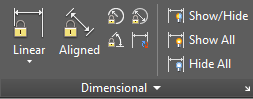# DIMCONSTRAINT (Command)

Applies dimensional constraints to selected objects or points on objects, or converts associative dimensions to dimensional constraints.Applies a dimensional constraint to a selected object or converts an associative dimension to a dimensional constraint.

Note: The L option (last object drawn) is not allowed in the DIMCONSTRAINT command as the constraint behavior is dependent on where you pick the object.

The following table outlines the valid constraint points for an object.

Objects

Valid Constraint Points

Line

Endpoints, Midpoint

Arc

Center, Endpoints, Midpoint

Spline

Endpoints

Ellipse, Circle

Center

Polyline

Endpoints, midpoints of line and arc subobjects, center of arc subobjects

Block, Xref, Text, Mtext, Attribute, Table

Insertion point

After you specify the dimensional constraint type, you can either enter an expression value or accept the default (constraintname=value).

The DIMCONSTRAINT command gives the same options as the following commands:

Linear (DCLINEAR)
Creates a horizontal, vertical, or rotated constraint based on the locations of the extension line origins and the dimension line.
Horizontal (DCHORIZONTAL)
Constrains the X distance between points on an object, or between two points on different objects.
Vertical (DCVERTICAL)
Constrains the Y distance between points on an object, or between two points on different objects.
Aligned (DCALIGNED)
Constrains the distance between two points on different objects.
Angular (DCANGULAR)
Constrains the angle between line or polyline segments, the angle swept out by an arc or a polyline arc segment, or the angle between three points on objects.